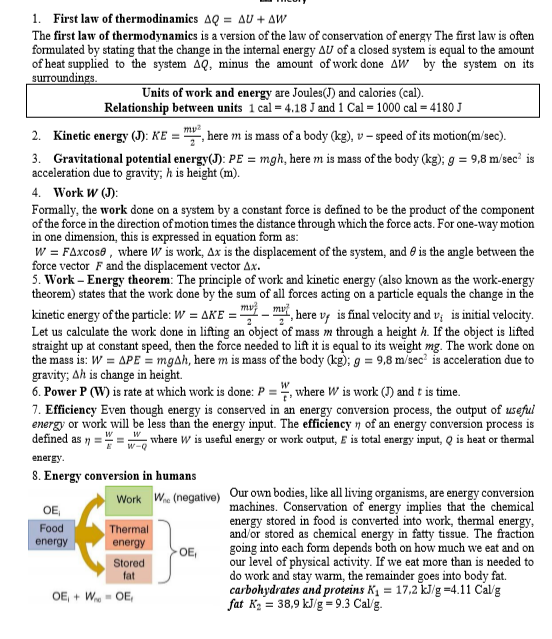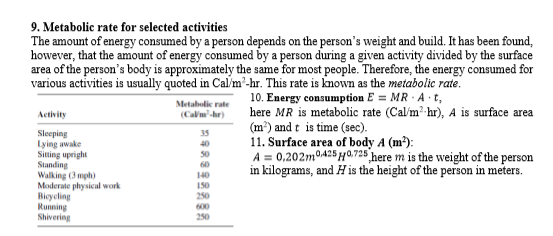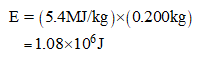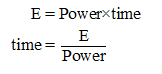# 1. First law of thermodinamics AQ = AU + AWThe first law of thermodynamics is a version of the law of conservation of energy The first law is oftenformulated by stating that the change in the internal energy AU of a closed system is equal to the amountof heat supplied to the system AQ, minus the amount of work done Aw by the system on itssurroundings.Units of work and energy are Joules(J) and calories (cal).Relationship between units 1 cal = 4.18 J and 1 Cal = 1000 cal = 4180 Jmy?Kinetic energy (J): KE = ", here m is mass of a body (kg), v – speed of its motion(m/sec).2.3. Gravitational potential energy(J): PE = mgh, here m is mass of the body (kg); g = 9,8 m/sec² isacceleration due to gravity; h is height (m).4.Work W (J):Formally, the work done on a system by a constant force is defined to be the product of the componentof the force in the direction of motion times the distance through which the force acts. For one-way motionin one dimension, this is expressed in equation form as:W = FAxcose , where W is work, Ax is the displacement of the system, and e is the angle between theforce vector F and the displacement vector Ax.5. Work – Energy theorem: The principle of work and kinetic energy (also known as the work-energytheorem) states that the work done by the sum of all forces acting on a particle equals the change in thekinetic energy of the particle: W = AKE = mvi – mvLet us calculate the work done in lifting an object of mass m through a height h. If the object is liftedstraight up at constant speed, then the force needed to lift it is equal to its weight mg. The work done onthe mass is: W = APE = mgAh, here m is mass of the body (kg); g = 9,8 m/sec' is acceleration due togravity; Ah is change in height.6. Power P (W) is rate at which work is done: P = ", where W is work (J) and t is time.7. Efficiency Even though energy is conserved in an energy conversion process, the output of usefulenergy or work will be less than the energy input. The efficiency 7 of an energy conversion process isdefined as n =4, here v¡ is final velocity and v;is initial velocity.where W is useful energy or work output, E is total energy input, Q is heat or thermalw-Qenergy.8. Energy conversion in humansWOur own bodies, like all living organisms, are energy conversionmachines. Conservation of energy implies that the chemicalenergy stored in food is converted into work, themal energy,and/or stored as chemical energy in fatty tissue. The fractiongoing into each form depends both on how much we eat and onour level of physical activity. If we eat more than is needed todo work and stay warm, the remainder goes into body fat.carbohydrates and proteins K, = 17,2 kJ/g =4.11 Cal/gfat K2 = 38,9 kJ/g = 9.3 Cal/g.(negative)WorkOE,FoodThermalenergyenergyOE,StoredfatOE, + W- OE, 9. Metabolic rate for selected activitiesThe amount of energy consumed by a person depends on the person's weight and build. It has been found,however, that the amount of energy consumed by a person during a given activity divided by the surfacearea of the person's body is approximately the same for most people. Therefore, the energy consumed forvarious activities is usually quoted in Cal/m-hr. This rate is known as the metabolic rate.10. Energy consumption E = MR · A t,here MR is metabolic rate (Calm? hr), A is surface area(m³) and t is time (sec).11. Surface area of body A (m?):A = 0,202m0425 H0,725 here m is the weight of the personin kilograms, and H is the height of the person in meters.Metabolic rate(Calm'-hr)ActivitySleepingLying awakeSiting uprightStandingWalking (3 mph)Moderate physical workBicyclingRunningShivering35405060140150250600250

Question
1 views

After eating a 200 g pottle of yoghurt, a person decides to go for a run to burn off the extra energy. The yoghurt provides 5.4 MJ kg−1, and he is able to raise his metabolic rate to 220W while running. How long does he have to run?help_outlineImage Transcriptionclose1. First law of thermodinamics AQ = AU + AW The first law of thermodynamics is a version of the law of conservation of energy The first law is often formulated by stating that the change in the internal energy AU of a closed system is equal to the amount of heat supplied to the system AQ, minus the amount of work done Aw by the system on its surroundings. Units of work and energy are Joules(J) and calories (cal). Relationship between units 1 cal = 4.18 J and 1 Cal = 1000 cal = 4180 J my? Kinetic energy (J): KE = ", here m is mass of a body (kg), v – speed of its motion(m/sec). 2. 3. Gravitational potential energy(J): PE = mgh, here m is mass of the body (kg); g = 9,8 m/sec² is acceleration due to gravity; h is height (m). 4. Work W (J): Formally, the work done on a system by a constant force is defined to be the product of the component of the force in the direction of motion times the distance through which the force acts. For one-way motion in one dimension, this is expressed in equation form as: W = FAxcose , where W is work, Ax is the displacement of the system, and e is the angle between the force vector F and the displacement vector Ax. 5. Work – Energy theorem: The principle of work and kinetic energy (also known as the work-energy theorem) states that the work done by the sum of all forces acting on a particle equals the change in the kinetic energy of the particle: W = AKE = mvi – mv Let us calculate the work done in lifting an object of mass m through a height h. If the object is lifted straight up at constant speed, then the force needed to lift it is equal to its weight mg. The work done on the mass is: W = APE = mgAh, here m is mass of the body (kg); g = 9,8 m/sec' is acceleration due to gravity; Ah is change in height. 6. Power P (W) is rate at which work is done: P = ", where W is work (J) and t is time. 7. Efficiency Even though energy is conserved in an energy conversion process, the output of useful energy or work will be less than the energy input. The efficiency 7 of an energy conversion process is defined as n = 4, here v¡ is final velocity and v; is initial velocity. where W is useful energy or work output, E is total energy input, Q is heat or thermal w-Q energy. 8. Energy conversion in humans W Our own bodies, like all living organisms, are energy conversion machines. Conservation of energy implies that the chemical energy stored in food is converted into work, themal energy, and/or stored as chemical energy in fatty tissue. The fraction going into each form depends both on how much we eat and on our level of physical activity. If we eat more than is needed to do work and stay warm, the remainder goes into body fat. carbohydrates and proteins K, = 17,2 kJ/g =4.11 Cal/g fat K2 = 38,9 kJ/g = 9.3 Cal/g. (negative) Work OE, Food Thermal energy energy OE, Stored fat OE, + W- OE, fullscreenhelp_outlineImage Transcriptionclose9. Metabolic rate for selected activities The amount of energy consumed by a person depends on the person's weight and build. It has been found, however, that the amount of energy consumed by a person during a given activity divided by the surface area of the person's body is approximately the same for most people. Therefore, the energy consumed for various activities is usually quoted in Cal/m-hr. This rate is known as the metabolic rate. 10. Energy consumption E = MR · A t, here MR is metabolic rate (Calm? hr), A is surface area (m³) and t is time (sec). 11. Surface area of body A (m?): A = 0,202m0425 H0,725 here m is the weight of the person in kilograms, and H is the height of the person in meters. Metabolic rate (Calm'-hr) Activity Sleeping Lying awake Siting upright Standing Walking (3 mph) Moderate physical work Bicycling Running Shivering 35 40 50 60 140 150 250 600 250 fullscreen
check_circle

Step 1

Given,

Energy provided by the yoghurt = 5.4MJkg-1

Step 2

The energy taken by the person isStep 3

We know,...

### Want to see the full answer?

See Solution

#### Want to see this answer and more?

Solutions are written by subject experts who are available 24/7. Questions are typically answered within 1 hour.*

See Solution
*Response times may vary by subject and question.
Tagged in

### Physics